Courses

# First Law Of Thermodynamics MCQ Level - 2 (Part - 2)

## 10 Questions MCQ Test Basic Physics for IIT JAM | First Law Of Thermodynamics MCQ Level - 2 (Part - 2)

Description
This mock test of First Law Of Thermodynamics MCQ Level - 2 (Part - 2) for Physics helps you for every Physics entrance exam. This contains 10 Multiple Choice Questions for Physics First Law Of Thermodynamics MCQ Level - 2 (Part - 2) (mcq) to study with solutions a complete question bank. The solved questions answers in this First Law Of Thermodynamics MCQ Level - 2 (Part - 2) quiz give you a good mix of easy questions and tough questions. Physics students definitely take this First Law Of Thermodynamics MCQ Level - 2 (Part - 2) exercise for a better result in the exam. You can find other First Law Of Thermodynamics MCQ Level - 2 (Part - 2) extra questions, long questions & short questions for Physics on EduRev as well by searching above.
QUESTION: 1

### Two moles of an ideal monoatomic gas is taken through a cycle ABCA as shown in the p-T diagram. During the process AB, Pressure and temperature of the gas vary such that pT = constant. If T1 = 300K then the work done on the gas in the process AB is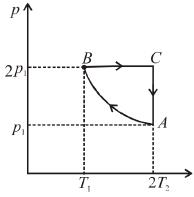Select one:

Solution:

Number of moles, n = 2, T1= 300 K, T2 =  2T1 = 600 K
During process A → B
pT =  constant
or p2V = constant = k [∴ pV = nRT]
p2V = k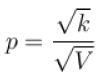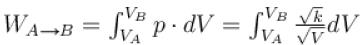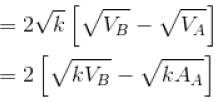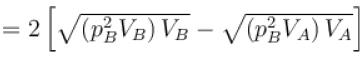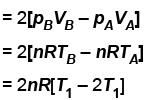= 2 × 2 × R × [300 – 600] = -1200 R
The correct answer is: –1200 R

QUESTION: 2

### One mole of a perfect gas is compressed adiabatically. The initial pressure and volume of the gas are 105N/m and 6L respectively. The final volume of the gas is 2L, molar specific heat of the gas at constant volume is 3R/2. The total work done is Select one:

Solution:

In adiabatic process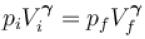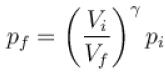Further,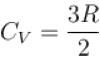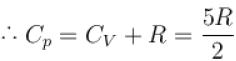or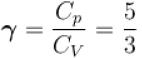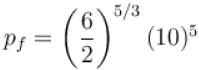= 6.24 × 105 N/m2
New york done in adiabatic process is given by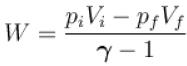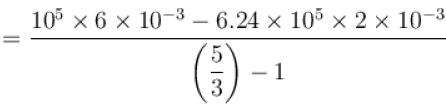= –972 J
The correct answer is: -972 J

QUESTION: 3

### Two identical containers A and B with frictionless pistons contains the same ideal gas at the same temperature and the same volume V. The mass of the gas in A is mA and that in B is mB . The gas in each cylinder is now allowed to expand isothermally to the final volume 2V. The changes in the pressure in A and B are found to be Δp and 1.5 Δp respectively. Then Select one:

Solution:

Process is isothermal. Therefore, T = constant.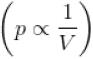volume is increasing, therefore, pressure will decrease.
In chamber A, as Pressure decrease,
So,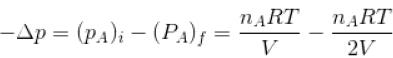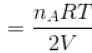...(i)
Similarly, in chamber B→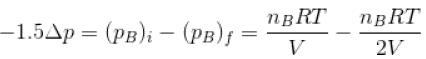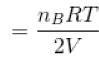...(ii)
From equation (1) and (2)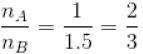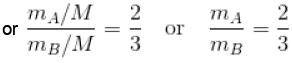or  2mA = 2mA
The correct answer is: 3 mA = 2 mB

QUESTION: 4

A cyclic process ABCA shown in the V-T diagram is performed with a constant mass of an ideal gas. Show the same process on a P-V diagram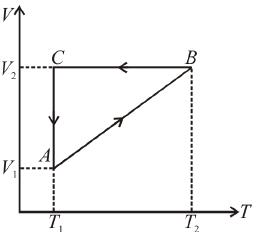Select one:

Solution:

Process A → B
From the graph,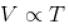From ideal gas equation, pV = nRT
⇒ p = constant
⇒ A → B, is isobaric process
So, in process A → B, when volume increases, pressure remains constant.
So, pV graph will be straight line parallel to V–axis as p = constant
Process B → C
From the graph, V = constant
From ideal gas equation, pV = nRT
⇒ pV = nRT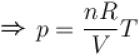[V is constant]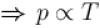But from the graph T is decreasing, so p also decrease.
But V remain constant.
pV graph will be straight line parallel to p-axis as V = constant
Process C → A
From the graph,
T = constant
From ideal gas equation, pV = nRT
⇒pV = nRT [T is constant]But from the graph, V decreases, so p should increase.
so, pV graph will be rectangular hyperbola as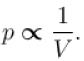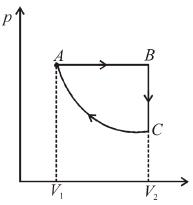QUESTION: 5

An ideal gas having initial pressure P, volume V and temperature T is allowed to expand adiabatically until its volume becomes 5.66V and its temperature falls to T/2. How many degree of freedom do the gas molecules have?

Solution:

In adiabatic process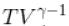= constant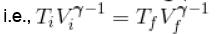Here,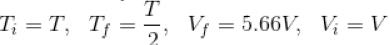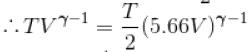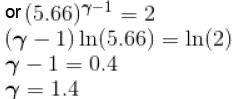We know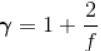Here f = degree of freedom
So, putting the value of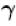f = 5

QUESTION: 6

An ideal gas has specific heat at constant pressure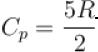The gas is kept in a closed vessel of volume 0.00833m , at a temperature of 300K and a pressure of 1.6 × 106N/m2. An amount of 2.49 × 104J of heat energy is supplied to the gas.
The final pressure and temperature of the gas are
Select one:

Solution:

Vessel is closed. Therefore, ΔW = 0
From first law of thermodynamics and ideal gas equation.
Q = ΔU + W
and  pV = nRT
∴ W = 0
⇒ Q = ΔU
Also, Δ = nCVΔT
or,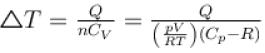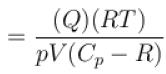Substituting the values, we have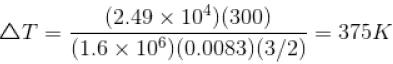ΔT = 375K
Tf = ΔT + T = 675K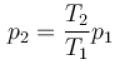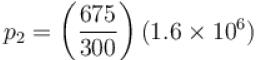= 3.6 × 106N/m2
The correct answer is: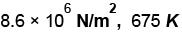QUESTION: 7

A monoatomic ideal gas, initially at temperature T1, is enclosed in a cylinder fitted with a frictionless piston. The gas is allowed to expand adiabatically to a temperature Tby releasing the piston suddenly. If L1 and L2 are the lengths of the gas column before and after expansion respectively, then T1 /T2 is given by
Select one:

Solution: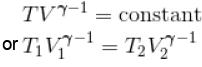For a monoatomic gas,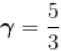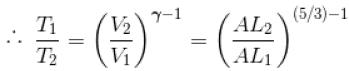(A = Area of cross-section of piston)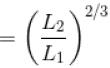The correct answer is: (L2 /L1)2/3

QUESTION: 8

p-V plots for two gas during adiabatic process are shown in the figure. Plots 1 and 2 should correspond respectively to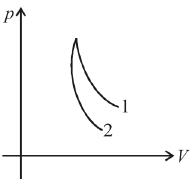Select one:

Solution:

Slope of p-V graph,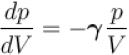slope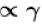(with negative sign)
From the given graph,
(slope)2 > (slope)1
∴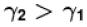Therefore, 1 should corresponding to O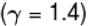and 2 should correspond to He (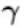= 1.67)
The correct answer is: He and O2

QUESTION: 9

Two cylinders A and B fitted with pistons contain equal amounts of an ideal diatomic gas at 300 K. The pistons of A is free to move, while that of B is kept fixed. The same amount of heat is given to the gas in each cylinder. If the rise in temperature of the gas in A is 30 K, then the rise in temperature of the gas in B is
Select one:

Solution:

A is free to move, therefore, heat will be supplied at constant pressure
∴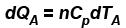...(i)
B is held fixed, therefore, heat will be supplied at constant volume.
∴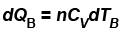...(ii)
But dQA = dQB  (given)
∴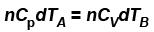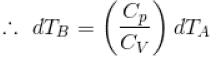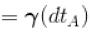[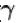= 1.4 (diatomic)]  (dTA = 30 K)
= (1.4) (30 K)
dTB = 42 K
The correct answer is: 42 K

QUESTION: 10

An ideal gas is taken through the cycle  A → B → C → A  a shown in the figure. If the net heat supplied to the gas in the cycle is 5J, the work done by the gas in the process  C → A  is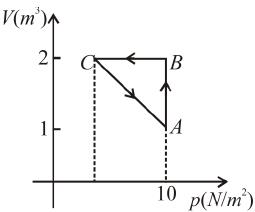Solution: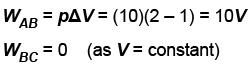From first law of thermodynamics
Q = W + ΔU
ΔU = 0     (process ABCA is cyclic)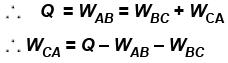= 5 – 10 – 0 = –5 J
The correct answer is: –5 J